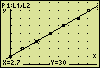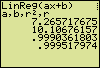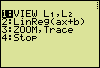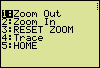# Linear Regressiona program for the Texas Instruments TI-83and TI-84 (Plus) graphing calculators

### Summary

This program is a shortened version of the program Regression in which more regression models are integrated.

Every measurement is influenced by many uncertainties. Measurement uncertainties can never be completely eliminated, so the true value for any quantity is never known exactly.
If two physical quantities are known to have a linear relationship, experimental data, when plotted on a graph, are expected to fit a straight line in a more or less accurate and precise way. Due to errors in the measuring process, not all the data fall exactly on the drawn line.Linear regression is a statistical tool that helps you to determine the "best-fitting" straight line for any given set of experimental data. In the relationship  y = ax + b  the constants a and b are being determined. The obtained function hands over which value is to be expected on an average for the one variable (y) at a given value of the other variable (x).You might prefer knowing more about linear regression beforehand. If so, it is recommended to view an interactive demonstration in order to understand the basic outcomes a linear regression analysis has to offer.

### Program Listing

Help
PROGRAM:REGLIN R : ALPHA, R keys
ClrHome > PRGM > I/O > ClrHome
RectGC:CoordOn > 2nd FORMAT > ...
: : ALPHA, : keys
GridOn:AxesOn
LabelOn:ExprOn
PlotsOff > 2nd STAT PLOT > PlotsOff
FnOff > VARS > Y-VARS > On/Off... > ...
GraphStyle(1,1) > PRGM > CTL > GraphStyle
, : comma key
1 → Xres "→" Symbolizes the STO> key
> VARS > Window... > Xres
Disp "STORE VALUES","INTO L1 AND L2." > PRGM > I/O > Disp
Space: ALPHA, .. (above 0 key)
L1 : 2nd, L1 keys
Pause > PRGM > CTL > Pause
(max(L1)–min(L1))/5 → U > MATH > NUM > max(
10^(int(log(U))) → Z 10^( : 2nd, 10x keys
> MATH > NUM > int(
U/Z → U
(int(√(U–1))²+1)Z → Xscl √( : 2nd, √ keys (square root)
² : x² key
> VARS > Window... > X/Y > Xscl
(max(L2)–min(L2))/5 → V
10^(int(log(V))) → Z
V/Z → V
(int(√(V–1))²+1)Z → Yscl √( : square root
Xscl and Yscl will be set to 1, 2, or 5 times a power of ten.
Plot1(Scatter,L1,L2, §) > 2nd STAT PLOT > Plot1(
> 2nd STAT PLOT > TYPE > Scatter
> 2nd STAT PLOT > MARK > § (small square)
ZoomStat > ZOOM > ZoomStat
ZoomSto > ZOOM > MEMORY > ...
Lbl 0 : ClrHome > PRGM > CTL > Lbl
> PRGM > I/O > ClrHome
Menu("","VIEW L1,L2",1, "LinReg(ax+b) ", 2, "ZOOM,Trace", 60, "Stop",7) > PRGM > CTL > Menu(
> STAT > CALC > LinReg(ax+b)
> TRACE
> PRGM > CTL > Stop
Lbl 60
Menu("","Zoom Out",61, "Zoom In",62, "RESET ZOOM",63, "Trace",64, "HOME",65) > ZOOM > ...
Lbl 1
Output(1,1,L1) > PRGM > I/O > ...
Pause :ClrHome
Output(1,1,L2)
Pause
Goto 0 > PRGM > CTL > ...
Lbl 2
Disp "LinReg(ax+b) ", "a,b,r²,r" > STAT > CALC > LinReg(ax+b)
> VARS > Statistics... > EQ > a (and b,r²,r)
LinReg(ax+b) Y1 > VARS > Y-VARS > Function... > Y1
Disp a,b,r²,r > VARS > ...
Pause
DispGraph > PRGM > I/O > DispGraph
Pause
Goto 0
Lbl 61
√(2) → Z : Z → XFact (square root)
Z → YFact > VARS > Window... > X/Y > Xfact
10^(int(log((Xmax-Xmin)/Z))) → Xscl
10^(int(log((Ymax-Ymin)/Z))) → Yscl
Zoom Out > ZOOM > Zoom Out
Pause
Goto 60
Lbl 62
Zoom In:Pause
Goto 60
Lbl 63
ZoomRcl:Pause > ZOOM > MEMORY > ZoomRcl
Goto 60
Lbl 64:Trace > TRACE
Goto 60
Lbl 65:Goto 0
Lbl 7
Xscl → ΔTbl:Stop > VARS > Table... > ΔTbl (Delta-Tbl)
> PRGM > CTL > Stop

### An Application

After putting in the program listing, you might like to see the program working. Try this example.

### The Experiment

A long tube is placed vertically and filled with a viscous liquid. (Which liquid is not?) When a bullet, being made up of steel, is let loose in the liquid, the sinking speed soon becomes constant. To determine this constant velocity, the position x is measured as a function of time t. The measuring outcome is:

 t(s) x(cm) 0,01,32,74,25,57,08,1 10203040506070

### Entering the Values

The time t is considered the independent variable and is measured along the horizontal axis of a graph. The position x, the dependent variable, is measured along the vertical axis. List 1 (L1) must contain values of the variable, which is to be plotted along the horizontal axis.
So, store the time values in List 1 and the position values in List 2 (L2). Here's how you can get access to these lists: > STAT > EDIT > Edit... > ...### Getting the Linear Equation and the Coefficient of Correlation

After preparing L1 and L2, run the program. A menu appears.
Select option "2:LinReg(ax+b)".
A fysical equivalent to the shown equation  y = ax + b  is the position equation
x(t) = v t  +  x(0).
The displayed value of the constant a in  y = ax + b  equals 7.26571..., meaning the constant velocity v amounts to 7,27 cm/s.
The position at the beginning of the measurements is
x(0) = = 10,1 cm.
Substituting the numerical values in the position equation leads to the linear equation in demand:
x(t) = 7,27 t  +  10,1  with x expressed in cm.The produced value of the coefficient of correlation (r = 0,99952) indicates that the found linear equation is only a fair representation of the relationship between the measured t and x values. Counting the nines, that directly follow the comma in the coefficient of correlation, is a convenient way to find out the usefulness of the equation. In chemical and physical determinations the number of nines may be interpreted in this way: five nines mean an excellent representation, four nines a good one, three nines a fair one, two nines a poor one, and one nine means a very poor representation. With no nines directly behind the comma the equation is "unusable".

### Viewing the Plotted Points and the Straight Line

Press ENTER.### Tracing

Once the graph has been drawn, press ENTER, select option "3:ZOOM,Trace" in the menu, and "4:Trace" in the following sub-menu. Next, use the left and right arrow keys to trace the values of the plotted measuring points.Press the up arrow key once to enable tracing the plotted line. Again, use the left and right arrow keys to explore the coordinates of some points of the line.Using the TABLE function is another way to get these values. Stop the execution of the program by selecting "5:HOME" in the sub-menu and "4:Stop" in the main menu. Then call on the TABLE function. You may have to adjust the table settings using the TBLSET function.Hein ten Horn
Top | Programs Texas Instruments | Home